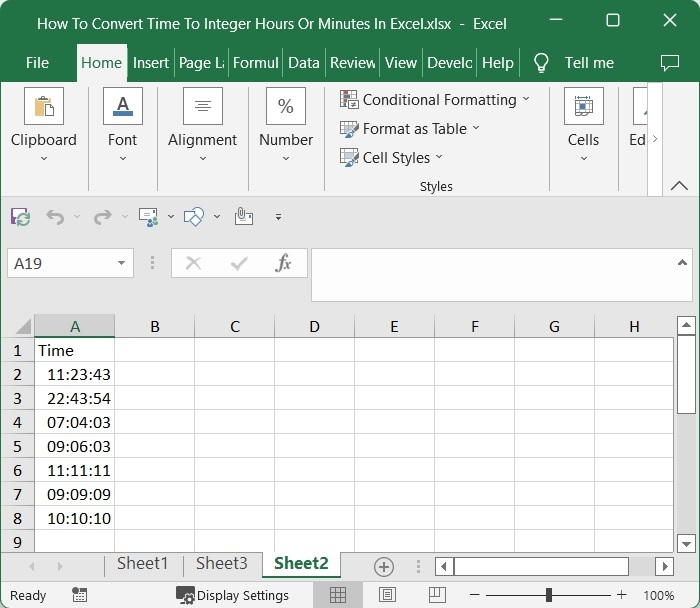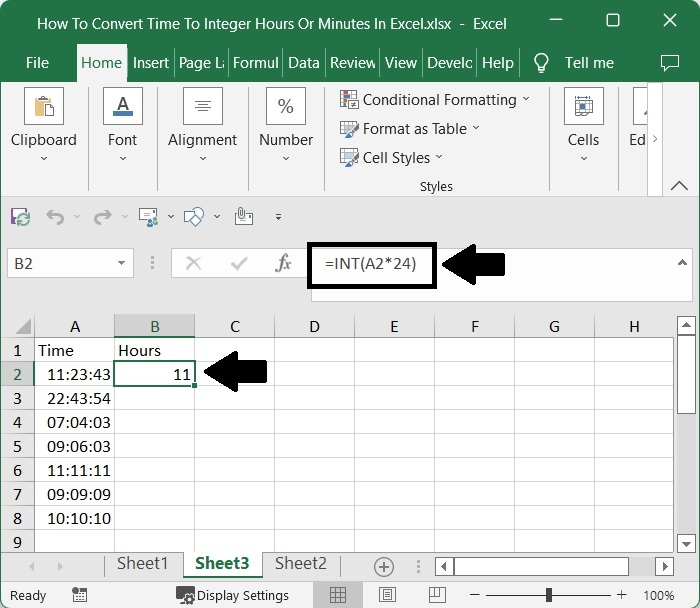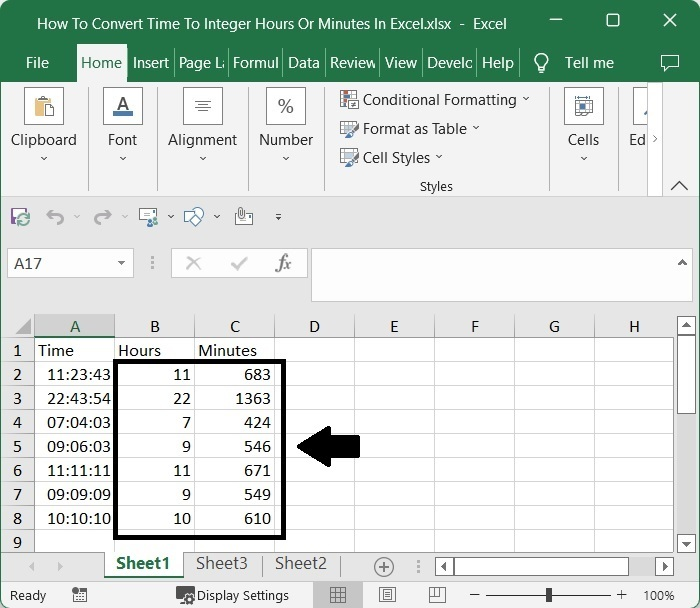# How to Convert Time to Integer Hours or Minutes in Excel?

An "Integer hour" is a time that is represented as a number. Sometimes in Excel, you may have needed to convert the given time to integer values. If we try to do this task manually, then it can be a time-consuming process, however we can solve this task using the formulas supported in Excel. The difference between a decimal and an integer is that the latter will not have any point values.

Read this tutorial to learn how you can convert time to integer hours or minutes in Excel. We must use the INT function because we must return the value as an integer rather than a decimal.

## Converting Time to Integer Hours in Excel

Here we will convert any one of the values using the int formula and then get all the results using the auto-fill handle. Let's go over a simple procedure for converting time to integer hours in Excel using various formulas.

### Step 1

Let us consider an Excel sheet where the data is a list of times similar to the one below.To get our first result, click on an empty cell, in this case cell B2, and enter the formula =INT(A2*24) and press Enter. In the formula, A2 is the address of our cell on the excel sheet, and we are multiplying with 24 because we have 24 hours in a day.

Empty cell > Formula > Enter### Step 2

Then, to get all the other results, drag down from the first result using the auto-fill handle, and our final result will be similar to the below image.To convert the time to integer minutes, we can use the formula =INT(A2*1440). We multiply by 1440 because one day is equal to 1440 minutes.

## Conclusion

In this tutorial, we used a simple example to demonstrate how you can convert time to integer hours in Excel.

Updated on: 06-Mar-2023

3K+ Views